### Home > CALC > Chapter 1 > Lesson 1.2.2 > Problem1-43

1-43.
1. Copy the graph below. Then complete it so it will have the symmetry described below. 1-43 HW eTool (Desmos). Homework Help ✎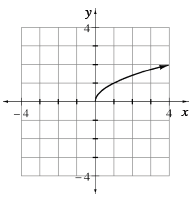1. Reflection symmetry across the y-axis.

2. Reflection symmetry across the x-axis.

3. Point symmetry at the origin. (This means a 180° rotation around the origin leaves the graph unchanged.)

4. Recall the definitions of even and odd functions. For each drawing, state if it is even, odd or neither.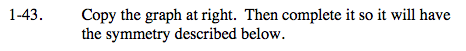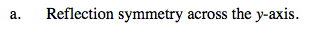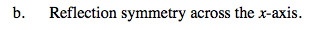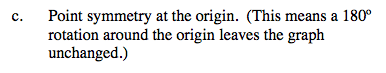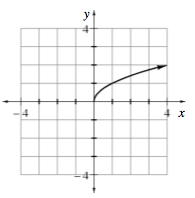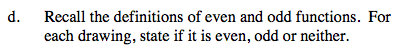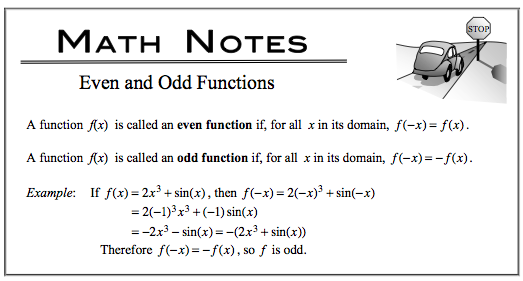Use the eTool below to view the graphs.
Click the link at right for the full version of the eTool: Calc 1-43 HW eTool